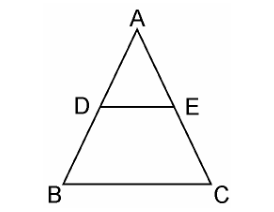Guru

# Using theorem 6.2 , prove that the line joining the mid-points of any two sides of a triangle is parallel to the third side. (Recall that you have done it in Class IX). Q.8

• 0

What is the best way to solve the problem of exercise 6.2 of triangles chapter of math, how i solve this problem because it is very important Using theorem 6.2 , prove that the line joining the mid-points of any two sides of a triangle is parallel to the third side. (Recall that you have done it in Class IX).

Share

1. Given, in ΔABC, D and E are the mid points of AB and AC respectively, such that,We have to prove that: DE || BC.

Since, D is the midpoint of AB

Also given, E is the mid-point of AC.

∴ AE=EC

⇒ AE/EC = 1

From equation (i) and (ii), we get,